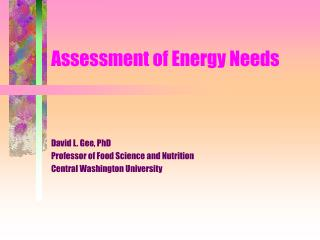# Assessment of Energy Needs - PowerPoint PPT PresentationDownload PresentationAssessment of Energy Needs

Assessment of Energy Needs
Download Presentation## Assessment of Energy Needs

- - - - - - - - - - - - - - - - - - - - - - - - - - - E N D - - - - - - - - - - - - - - - - - - - - - - - - - - -
##### Presentation Transcript

1. Assessment of Energy Needs David L. Gee, PhD Professor of Food Science and Nutrition Central Washington University

2. Reasons to assess energy needs • Energy needs are highly variable • Prevent underfeeding • decrease organ mass and function • impaired wound healing • impaired immune response • Prevent overfeeding • excessive CO2 production • Respiratory acidosis • Hyperglycemia and insulin resistance • fluid retention and fat gain (fatty liver)

3. Estimation of Resting Energy Expenditure (REE) with Prediction Equations • Harris-Benedict Equation (1919) • based on gender, weight, height, age • WHO Equations (1982) • based on gender, weight, age • Errors in estimation: • Standard deviation = 10% • 95% confidence interval = 20%

4. Validation of Several Established Equations for Resting Metabolic Rate in Obese and Nonobese People.Frankenfield et al., JADA 103:1152(2003) • 130 healthy adults (BMI=18.8-96.8) • 98% white • Compared equations to indirect calorimetry • Harris-Benedict • Adjusted Harris-Benedict (25% of excess wt) • Owen (1986) • Mifflin (1990) • Men: kcal/d=5+10(wt)-6.25(ht)-5(age) • Women: kcal/d=-161+10(wt)+6.25(ht)-5(age) • Wt=kg, ht=cm,age=yrs

5. Accurate Determination of Energy Needs in Hospitalized Patients.Boullata et al., JADA 107:-393-401 (2007) • 395 hospitalized patients • Compared prediction equations against measured REE • Harris-Benedict, Mifflin, 6 others • Conclusions: • Most accurate was Harris-Benedict multiplied by 1.1, but only 62% were within 10% of measured REE • “No equation accurately predicted REE in most hospitalized patients … only indirect calorimetry will provide accurate assessment of energy needs.”

6. Why prediction equations fail… • Equations based on gender, height, weight and age explain ~ 80% of individual variation in REE • Sources of other variations • Mass of various tissues • Visceral tissues 10x more active than muscle tissue at rest and 100x more active than adipose • Knowing body composition based on 2-component or 4-component models still inadequate

7. Estimation of Total Energy Expenditure is even less accurate • TEE = REE + Activity + TEF + Injury factors • estimations of • activity • TEF • injury factors • are crude estimates

8. Indirect Calorimetry • Estimation of energy expenditure based on respiratory gases • oxygen consumed • carbon dioxide produced • Nutrient + O2 -> CO2 + H2O + energy • Metabolic Carts • Hand-held Indirect Calorimeters

9. Oxidation of glucose • Glucose + 6O2 -> 6CO2 + 6H2O + 673Cal/mol • 673/6 = 112 Cal/mol O2 • Respiratory Quotient (RQ) = Respiratory Exchange Ratio (RER) = CO2/O2 • RQCHO = 6/6 = 1.0

10. Oxidation of Fat • Palmitate + 23O2 -> 16CO2 + 16H2O + 2398Cal/mol • 2398/23 = 104 Cal/mol O2 • RQ = 16/23 = 0.7

11. Oxidation of Amino Acids • RQ for amino acids and the energy produced per mol of O2 varies for each amino acid • RQ for average protein is 0.85 • Contribution of protein oxidation is ignored because: • small compared to fat and glucose • RQ at rest is typically close to 0.85 • protein oxidation during short-term exercise is very small compared to fat and glucose • To measure protein oxidation, one needs to collect 24hr urine to measure total urea production

12. RQ (RER) Tables • RQ or RER can be used to: • Determine the calories burned per • liter of oxygen consumed or • Liter of carbon dioxide produced • Determine the % of calories produced by burning fats and carbohydrates

13. Indirect Calorimetry CalculationsMethod I (rough estimate) • Approximately 5.0 Cal/l O2 • l O2/min x 5.0 Cal/lO2 = Cal/min • example: • VO2 = volume of O2 consumed/min = 0.2 l/min • then 0.2 x 5 = 1 Cal/min • if REE, then 1 Cal/min x 1440 min/d = 1440Cal/d

14. Indirect Calorimetry CalculationsMethod 2 (not so rough estimate) • More accurately: 4.8 Cal/l O2 • Example • if: VO2 = 0.2 l/min • then: 0.2 x 4.8 = 0.96 Cal/min • if REE, then 0.96 x 1440 = 1382 Cal/d

15. Indirect Calorimetry CalculationsMethod 3 - using total RQ • if VO2 = 0.2 l/min and VCO2 = 0.17 l/min • then RQ = 0.17 / 0.2 = 0.85 • if RQ = 0.85, then 4.862 Cal/lO2 • 0.2 x 4.862 = 0.97 Cal/min • 0.97 x 1440 = 1400 Cal/day

16. Determination of VO2 and VCO2 • Go to the Word document on Indirect Calormetry Calculations